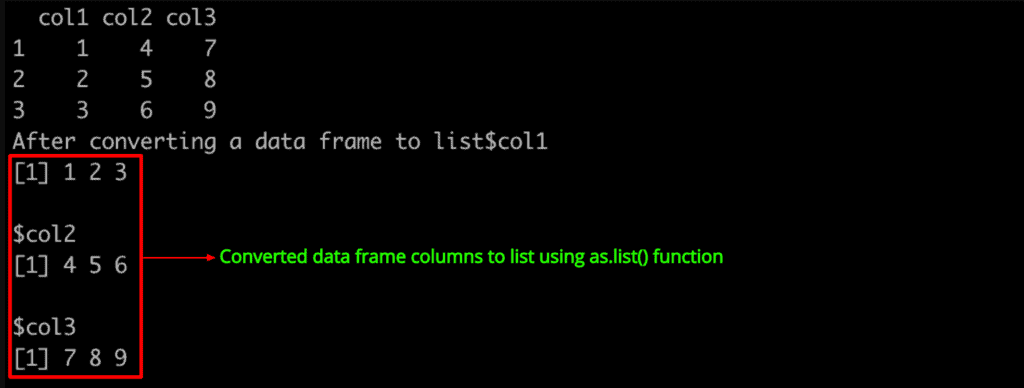# How to Use as.list() Function in R

The as.list() function in R is used to convert an object to a list. These objects can be vectors, matrices, data frames, and factors.

## Syntax

``as.list(object)``

## Parameters

It takes an object as a parameter, a vector, matrix, factor, or data frame.

## Example 1: Converting vector to a list

When you apply as.list() function to a vector, each element becomes an individual component of the list.

``````vec <- 1:5
vec

cat("After converting a vector to list", "\n")

lst <- as.list(vec)
lst
``````

Output

`````` 1 2 3 4 5

After converting a vector to list

[]
 1

[]
 2

[]
 3

[]
 4

[]
 5
``````

## Example 2: Converting data frame to list

To convert a data frame to a list in R using the as.list() function, each column of the data frame is converted into a separate element of the list. Each element of the list will be a vector containing the data from one column of the data frame.

``````df <- data.frame(
col1 = c(1, 2, 3),
col2 = c(4, 5, 6),
col3 = c(7, 8, 9)
)
df

lst <- as.list(df)
cat("After converting a data frame to list")
lst
``````

OutputThis is helpful for situations where you need to process or manipulate the columns of a data frame independently as vectors, or when you’re passing data frame columns to functions that require inputs as lists.

## Example 3: Converting matrix to list

To convert a matrix to a list in R using the as.list() function, you need to decide how you want the matrix elements to be organized within the list.

Unlike vectors or data frames, where the conversion is straightforward (each element or column becomes a list item), the structure of the resulting list from a matrix can vary based on your needs.

### Converting each column into a list element

This is similar to how columns of a data frame are converted. Each column of the matrix becomes a separate vector within the list.

``````mat <- matrix(1:9, nrow = 3, ncol = 3)

list_by_column <- as.list(as.data.frame(mat))

print(list_by_column)``````

Output

``````\$V1
 1 2 3

\$V2
 4 5 6

\$V3
 7 8 9``````

### Converting each row into a list element

Each row of the matrix becomes a separate vector within the list.

``````mat <- matrix(1:9, nrow = 3, ncol = 3)

list_by_row <- as.list(as.data.frame(t(mat)))

print(list_by_row)
``````

Output

``````\$V1
 1 4 7

\$V2
 2 5 8

\$V3
 3 6 9
``````

We converted each row of a matrix into a list element by transposing the matrix first and then used the as.list() function.

## Example 4: Converting a factor to a list

You can use the as.list() function to convert a factor to a list.

``````main_factor <- factor(c("apple", "banana"))

lst <- as.list(main_factor)

lst
``````

Output

``````[]
 apple
Levels: apple banana

[]
 banana
Levels: apple banana
``````

That’s it.